7.2: Operations with Vectors

•• Contributed by CK12
• CK12

When two or more forces are acting on the same object, they combine to create a new force.  A bird flying due south at 10 miles an hour in a headwind of 2 miles an hour only makes headway at a rate of 8 miles per hour.  These forces directly oppose each other.  In real life, most forces are not parallel.  What will happen when the headwind has a slight crosswind as well, blowing NE at 2 miles per hour.  How far does the bird get in one hour?

Basic Vector Operations

Scalar multiplication means to multiply a vector by a number. This changes the magnitude of the vector, but not its direction. If $$\vec{v}=<3,4>,$$ then $$2 \vec{v}=<6,8>.$$ Scalar multiplication is fairly simple.

Adding and subtracting vectors is slightly more difficult. When adding vectors, place the tail of one vector at the head of the other. This is called the tail-to-head rule. The vector that is formed by joining the tail of the first vector with the head of the second is called the resultant vector.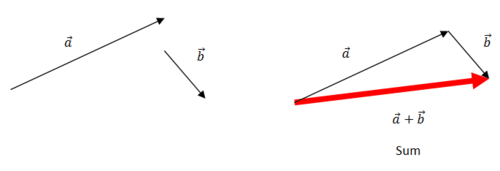Vector subtraction reverses the direction of the second vector. $$\vec{a}-\vec{b}=\vec{a}+(-\vec{b})$$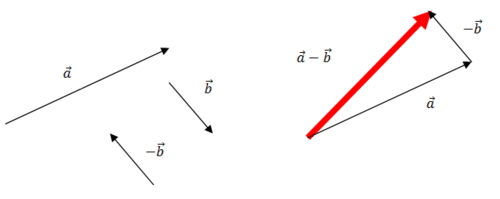Adding vectors can be done in either order (just like with regular numbers). Subtracting vectors must be done in a
specific order or else the vector will be negative (just like with regular numbers).

To find the length or magnitude of a resultant vector, you can use the law of cosines. To do this, you also need to know the angle between the two vectors. Say you were given two vectors $$\vec{a}$$ and $$\vec{b}$$, have magnitudes of 5 and 9 respectively and that the angle between the vectors is $$53^{\circ}$$. To find the magnitude of $$\vec{a}+\vec{b}$$, which is written as $$|\vec{a}+\vec{b}|,$$ notice that you have a parallelogram.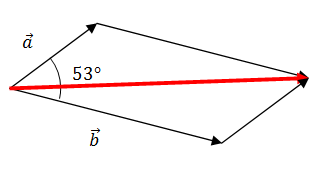In order to fine the magnitude of the resulting vector in red, note that the triangle on the bottom has sides 9 and 5 with included angle $$127^{\circ}$$ due to the properties of parallelograms. And, so applying the Law of cosines, you get:

\begin{aligned} x^{2} &=9^{2}+5^{2}-2 \cdot 9 \cdot 5 \cdot \cos 127^{\circ} \\ x & \approx 12.66 \end{aligned}

For this video, focus on scalar multiplication and adding and subtracting vectors:

Examples

Example 1

Earlier, you were asked about how fast a bird was flying. A bird flying due south at 10 miles an hour with a cross headwind of 2 mph heading NE would have a force diagram that looks like this: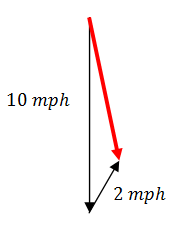The angle between the bird's vector and the wind vector is $$45^{\circ}$$ which means this is a perfect situation for the Law
of cosines. Let $$x=$$ the red vector.

\begin{aligned} x^{2} &=10^{2}+2^{2}-2 \cdot 10 \cdot 2 \cdot \cos 45^{\circ} \\ x & \approx 8.7 \end{aligned}

The bird is blown slightly off track and travels only about 8.7 miles in one hour.

Example 2

Using the vectors with magnitude 5 and 9 and angle of $$53^{\circ}$$ from the main section, what is the angle that the sum $$\vec{a}+\vec{b}$$ makes with $$\vec{a} ?$$

Start by drawing a good picture and labeling what you know. $$|\vec{a}|=5,|\vec{b}|=9,|\vec{a}+\vec{b}| \approx 12.66$$. since you know three sides of the triangle and you need to find one angle, this is the SSS application of the Law of cosines.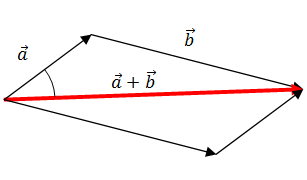\begin{aligned} 9^{2} &=12.66^{2}+5^{2}-2 \cdot 12.66 \cdot 5 \cdot \cos \theta \\ \theta &=34.6^{\circ} \end{aligned}

Example 3

Elaine started a dog walking business. She walks two dogs at a time named Elvis and Ruby. They each pull her in different directions at a $$45^{\circ}$$ angle with different forces. Elvis pulls at a force of $$25 \mathrm{~N}$$ and Ruby pulls at a force of $$49 N$$. How hard does Elaine need to pull so that she can stay balanced? Note that $$\mathrm{N}$$ stands for Newtons which is the standard unit of force.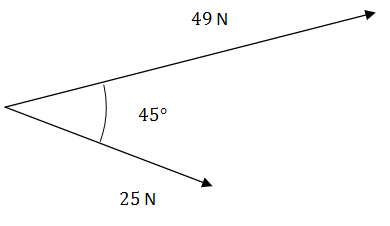Even though the two vectors are centered at Elaine, the forces are added which means that you need to use the tail-to-head rule to add the vectors together.  Finding the angle between each component vector requires logical use of supplement angles.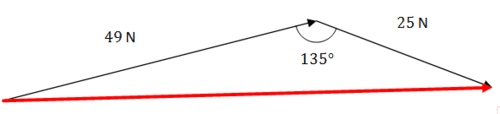\begin{aligned} x^{2} &=49^{2}+25^{2}-2 \cdot 49 \cdot 25 \cdot \cos 135^{\circ} \\ x & \approx 68.98 \mathrm{~N} \end{aligned}

In order for Elaine to stay balanced, she will need to counteract this force with an equivalent force of her own in the exact opposite direction.

Example 4

Consider vector $$\vec{v}=<2,5>$$ and vector $$\vec{u}=<-1,9>$$. Determine the component form of the following: $$3 \vec{v}-2 \vec{u}$$

Do multiplication first for each term, followed by vector subtraction.

\begin{aligned} 3 \cdot \vec{v}-2 \cdot \vec{u} &=3 \cdot<2,5>-2 \cdot<-1,9>\\ &=<6,15>-<-2,18>\\ &=<8,-3>\end{aligned}

Example 5

An airplane is flying at a bearing of $$270^{\circ}$$ at $$400 \mathrm{mph}$$. A wind is blowing due south at $$30 \mathrm{mph}$$. Does this cross wind affect the plane's speed?

Since the cross wind is perpendicular to the plane, it pushes the plane south as the plane tries to go directly east. As a result the plane still has an airspeed of 400 mph but the groundspeed (true speed) needs to be calculated.

\begin{aligned} 400^{2}+30^{2} &=x^{2} \\ x & \approx 401 \end{aligned}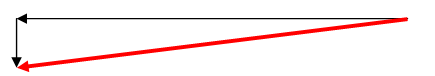Review

Consider vector $$\vec{v}=<1,3>$$ and vector $$\vec{u}=<-2,4>$$

1. Determine the component form of $$5 \vec{v}-2 \vec{u}$$.

2. Determine the component form of $$-2 \vec{v}+4 \vec{u}$$.

3. Determine the component form of $$6 \vec{v}+\vec{u}$$.

4. Determine the component form of $$3 \vec{v}-6 \vec{u}$$.

5. Find the magnitude of the resultant vector from #1.

6. Find the magnitude of the resultant vector from # 2 .

7. Find the magnitude of the resultant vector from $$\# 3$$.

8. Find the magnitude of the resultant vector from #4.

9. The vector $$<3,4>$$ starts at the origin. What is the direction of the vector?

10. The vector $$<-1,2>$$ starts at the origin. What is the direction of the vector?

11. The vector $$<3,-4>$$ starts at the origin. What is the direction of the vector?

12. A bird flies due south at 8 miles an hour with a cross headwind blowing due east at 15 miles per hour. How far does the bird get in one hour?

13. What direction is the bird in the previous problem actually moving?

14. A football is thrown at 50 miles per hour due north. There is a wind blowing due east at 8 miles per hour. What is the actual speed of the football?

15. What direction is the football in the previous problem actually moving?